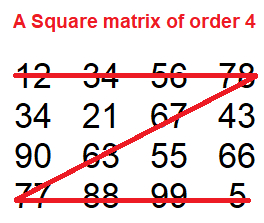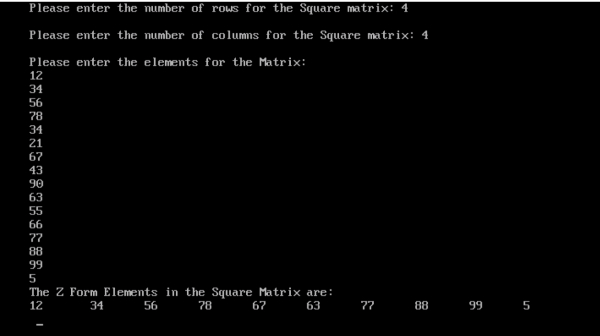# Program to Print the Squared Matrix in Z form in C

## Program Description

Print the elements of the squared matrix in Z form

A square matrix is a matrix with the same number of rows and columns. An n-by-n matrix is known as a square matrix of order

## AlgorithmTo print the elements of the Square Matrix in Z form
We need to print the first row of matrix then diagonal and then last row of the square matrix.

## Example

/* Program to print a square matrix in Z form */
#include<stdio.h>
int main(){
int rows, cols, r, c, matrix;
clrscr(); /*Clears the Screen*/
printf("Please enter the number of rows for the Square matrix: ");
scanf("%d", &rows);
printf("");
printf("Please enter the number of columns for the Square matrix: ");
scanf("%d", &cols);
printf("");
printf("Please enter the elements for the Matrix: ");
for(r = 0; r < rows; r++){
for(c = 0;c < cols;c++){
scanf("%d", &matrix[r][c]);
}
}
printf("The Z Form Elements in the Square Matrix are: ");
printf("");
for(r = 0; r < rows; r++){
printf("%d\t ", matrix[r]);
}
for (r=1,c=cols-2;r<cols && c>=0;r++,c--){
printf("%d\t ", matrix[r][c]);
}
for (r = 1; r < cols; r++){
printf("%d\t ", matrix[cols-1][r]);
}
getch();
return 0;
}

## OutputUpdated on: 09-Jan-2020

478 Views